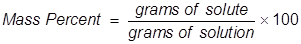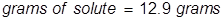# Mass Percent Formula

Mass Percent Formula

Mass percent is used as a way of expressing a concentration or a way of describing a component in a mixture. For a solution, the mass percent is expressed as the grams of solute divided by the grams of solution, then multiplied by 100 to get a percentage.Mass Percent Formula Questions:

1. Calculate the mass percent of sodium hypochlorite in commercial bleach, if 1.00 grams of NaOCl (the active ingredient in bleach) is dissolved in 19.05 grams of solution.2. Calculate the grams of NaOCl (5.25% by mass) in 245 grams of a commercial bleach solution.

In this problem the equation should be rearranged to solve for the grams of solute.Related Links: Force, Mass, Acceleration Quiz Mass vs. Weight